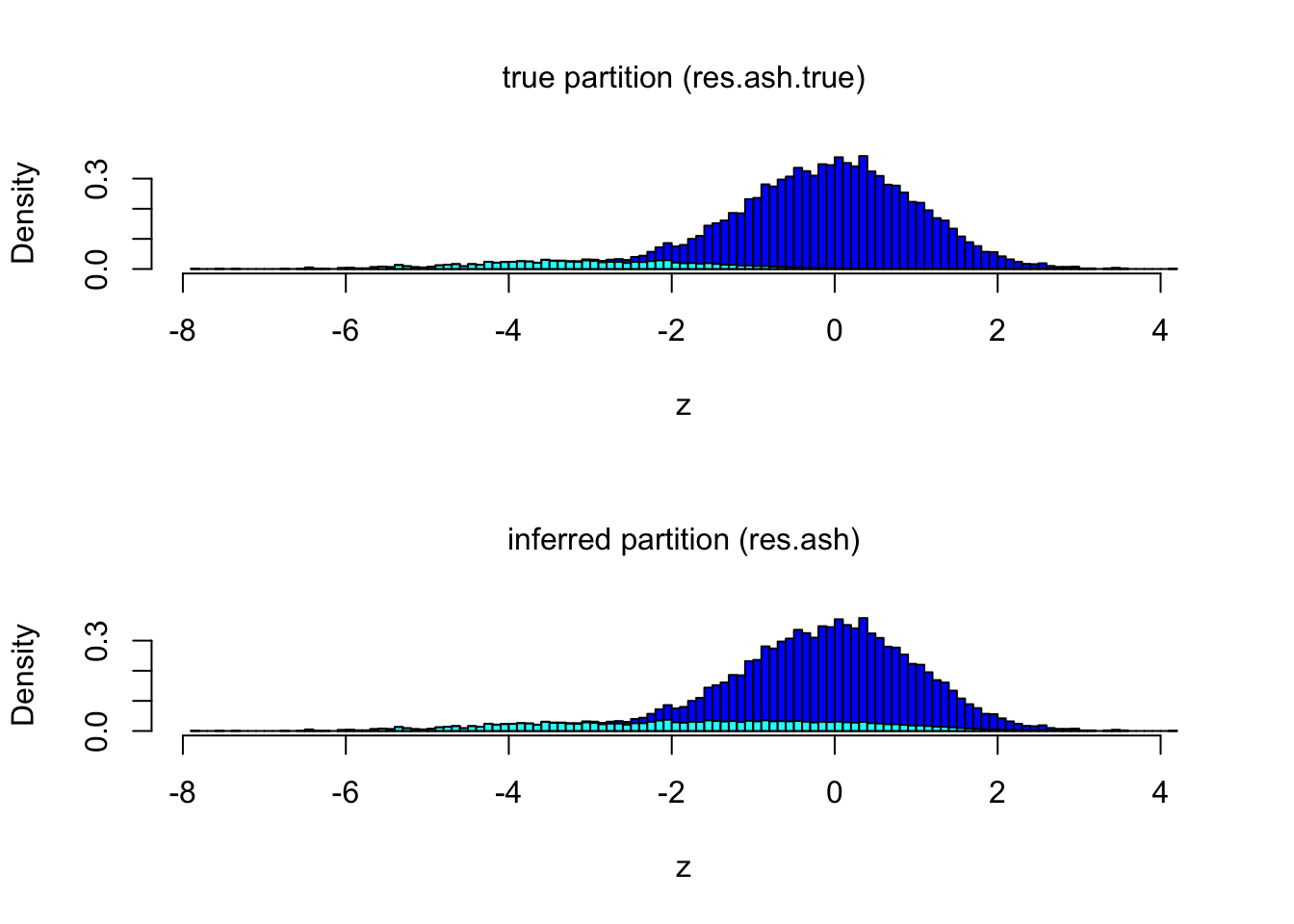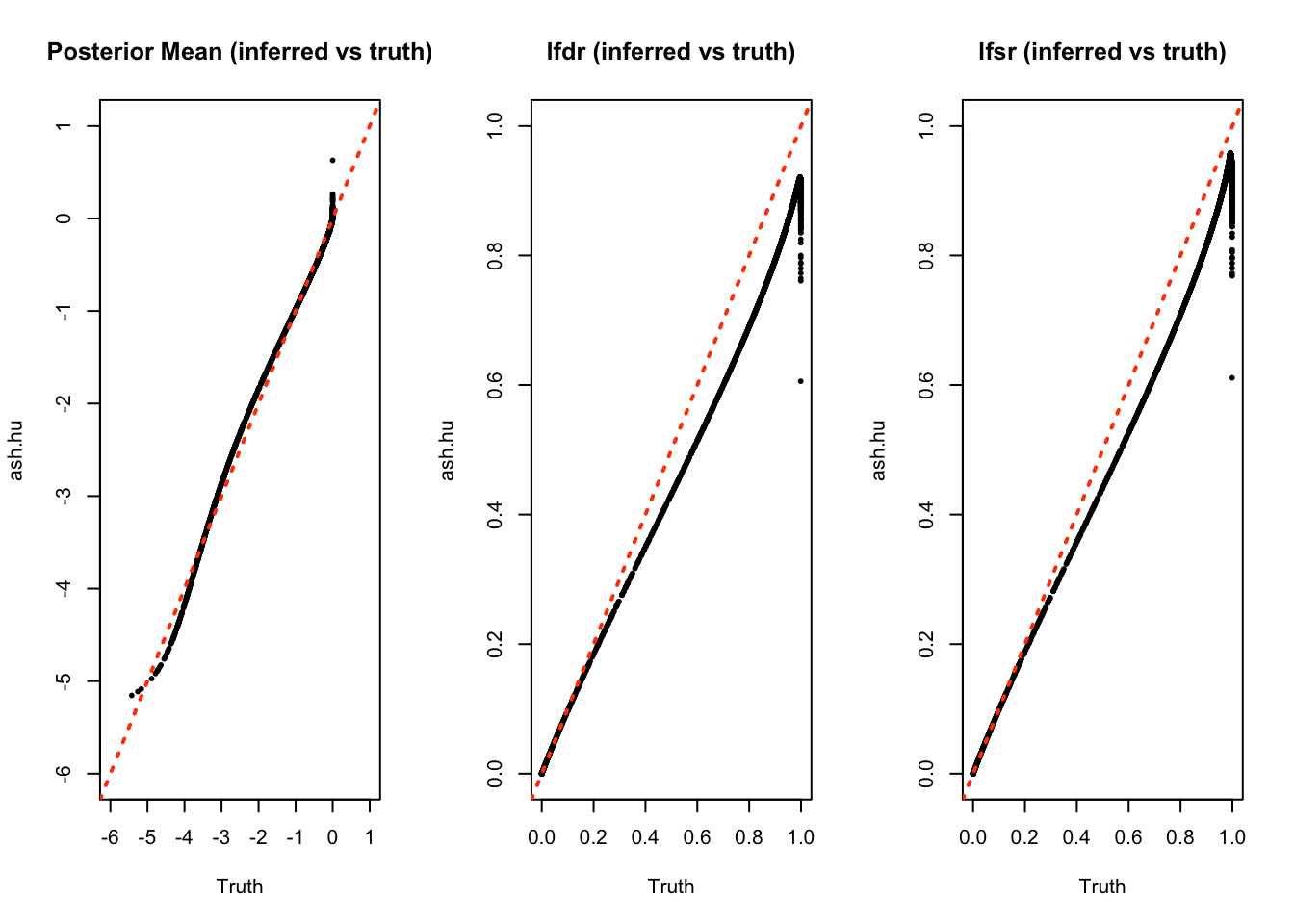Last updated: 2017-01-15

Code version: 94a3347615361216b39b8a9333bfa8354794e0ff

First, we load the necessary libraries.

``````library(ashr)
library(qvalue)
library(locfdr)``````

## Introduction

This example comes from Efron (2008) p16 when examining the false coverage rate (FCR). I selected this example because the distribution of the non-zero effects is highly assymetric and not at all unimodal at zero, both issues a referee asked me to elaborate on. Specifically, the distribution of the non-zero effects is N(-3,1). Here I simulate data, and apply ash (with the “halfuniform” option to allow for asymmetric g).

``````set.seed(10)
nsamp   = 1e4
altmean = -3
mu0     = rep(0,nsamp)
mu1     = rnorm(nsamp,altmean,1)
comp    = rbinom(nsamp,1,0.1)
mu      = ifelse(comp == 0,mu0,mu1)
z       = rnorm(nsamp,mu,1)

# Fit the model.
res.ash = ash(z,1,mixcompdist = "halfuniform")``````

We can also run ash with the “true” g, to allow us to compare the lfsr, lfdr, etc.

``````true.g       = normalmix(c(0.9,0.1),c(0,-3),c(0,1))
res.ash.true = ash(z,1,g = true.g,fixg = TRUE)``````

Here we can see how the partition of \(z\) scores compares with the truth. Note the effect of the unimodal assumption is to extend the inferred alternative distribution toward 0. Here, `nullalthist` plots a histogram of z scores, highlighting the alternative distribution of z scores that is implied by the localfdr values lfdr.

``````source("../R/nullalthist.R")
par(mfcol = c(2,1),cex.main = 1,font.main = 1)
nullalthist(z,lfdr = get_lfdr(res.ash.true),main = "true partition (res.ash.true)")
nullalthist(z,lfdr = get_lfdr(res.ash),main = "inferred partition (res.ash)")``````Comparing the inferred posterior means, lfdr, and lfsr with the true values of these quantities, we find reassuringly good correspondence.

``````par(mfrow = c(1,3))
plot(get_pm(res.ash.true),get_pm(res.ash),xlab = "Truth",ylab = "ash.hu",
main = "Posterior Mean (inferred vs truth)",pch = 20,cex = 0.6,
xlim = c(-6,1),ylim = c(-6,1))
abline(a = 0,b = 1,col = "orangered",lwd = 2,lty = "dotted")

plot(get_lfdr(res.ash.true),get_lfdr(res.ash),xlab = "Truth", ylab = "ash.hu",
main = "lfdr (inferred vs truth)",pch = 20,cex = 0.6,
xlim = c(0,1),ylim = c(0,1))
abline(a = 0,b = 1,col = "orangered",lwd = 2,lty = "dotted")

plot(get_lfsr(res.ash.true),get_lfsr(res.ash),xlab = "Truth", ylab = "ash.hu",
main = "lfsr (inferred vs truth)",pch = 20,cex = 0.6,
xlim = c(0,1),ylim = c(0,1))
abline(a = 0,b = 1,col = "orangered",lwd = 2,lty = "dotted")``````## Comparison with qvalue and locfdr

We can also run qvalue and locfdr. We see that locfdr perhaps performs a bit better than ash for the decomposition here, but the estimated local fdrs are pretty similar. Here qvalue does less well because of the asymmetry which it didn’t take account of.

``res.locfdr = locfdr(z,nulltype = 0)``